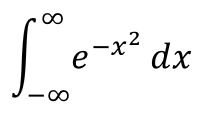## 单变量高斯积分- 阅读剩余部分 -

## 推导 Hubbard-Stratonovich 变换Nigel Goldenfeld

\begin{equation*} \int_{-\infty}^{\infty}\Pi_{i=1}^N\left(\frac{dx_i}{\sqrt{2\pi}}\right)\exp\left(-\frac{1}{2}x_iA_{ij}x_j+x_iB_i \right)=\frac{1}{\sqrt{\mathrm {det} A}}e^{\frac{1}{2}B_i(A^{-1})_{ij}B_j} \end{equation*}

- 阅读剩余部分 -